#### Chapter 12 Heron's Formula R.D. Sharma Solutions for Class 9th Exercise 12.2

Exercise 12.2

Q1. Find the area of a quadrilateral ABCD is which AB = 3 cm, BC = 4 cm, CD = 4 cm, DA = 5 cm and AC = 5 cm.

Solution

The quadrilateral ABCD having sides AB, BC, CD, DA and diagonal AC = 5 cm is given, where AC divides quadrilateral ABCD into two triangles ΔABC and ΔADC. We will find the area of the two triangles separately and them to find the area of quadrilateral ABCD.2. The sides of a quadrangular field, taken in order are 26 m, 27 m, 7 m are 24 m respectively. The angle contained by the last two sides is a right angle. Find its area.

Solution

We assume quadrilateral ABCD be the quadrangular field having sides AB, BC, CD, DA and ∠CDA = 90°.
We take a diagonal AC, where AC divides quadrilateral ABCD into two triangles ΔABC and ΔADC We will find the area of two triangles ΔABC and ΔADC separately and add them to find the area of the quadrangular ABCD.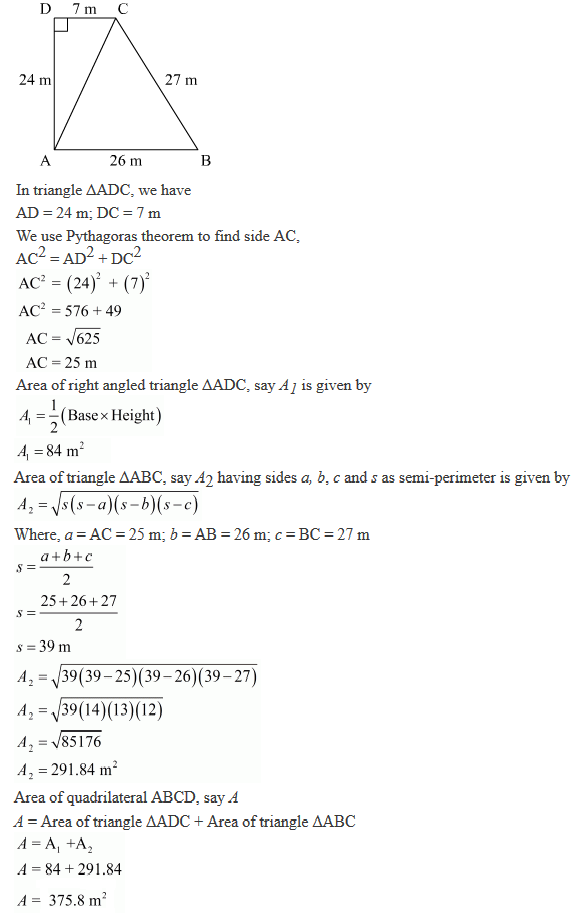3. The sides of a quadrilateral, taken in order are 5, 12, 14 and 15 metres respectively, and the angle contained by the first two sides is a right angle. Find its area.

Solution
We assume ABCD be the quadrilateral having sides AB, BC, CD, DA and angle ∠ABC = 90°.
We take a diagonal AC, where AC divides quadrilateral ABCD into two triangles ΔABC and ΔADC. We will find the area of these two triangles and add them to find the area of the quadrilateral ABCD4. A park, in the shape of a quadrilateral ABCD, has ∠C = 90°, AB = 9 m, BC = 12 m, CD= 5 m and AD = 8 m. How much area does it occupy?

Solution
We assume ABCD be the quadrilateral having sides AB, BC, CD, DA and ∠DCB = 90°.
We take a diagonal DB, where DB divides ABCD into two triangles ΔBCD and ΔAB5. Two parallel side of a trapezium are 60 cm and 77 cm and other sides are 25 cm and 26 cm. Find the area of the trapezium.

Solution
We assume ABCD be the given trapezium where AB is parallel to DC.
We draw CE parallel to AD from point C.
Therefore, a parallelogram ADCE is formed having AD parallel to CE and DC parallel to AE.
AE = 60 cm; CE = 25 cm; BE = AB-AE = 17 cm
Basically we will find the area of the triangle BCE and area of the parallelogram AECD and add them to find the area of the trapezium ABCD.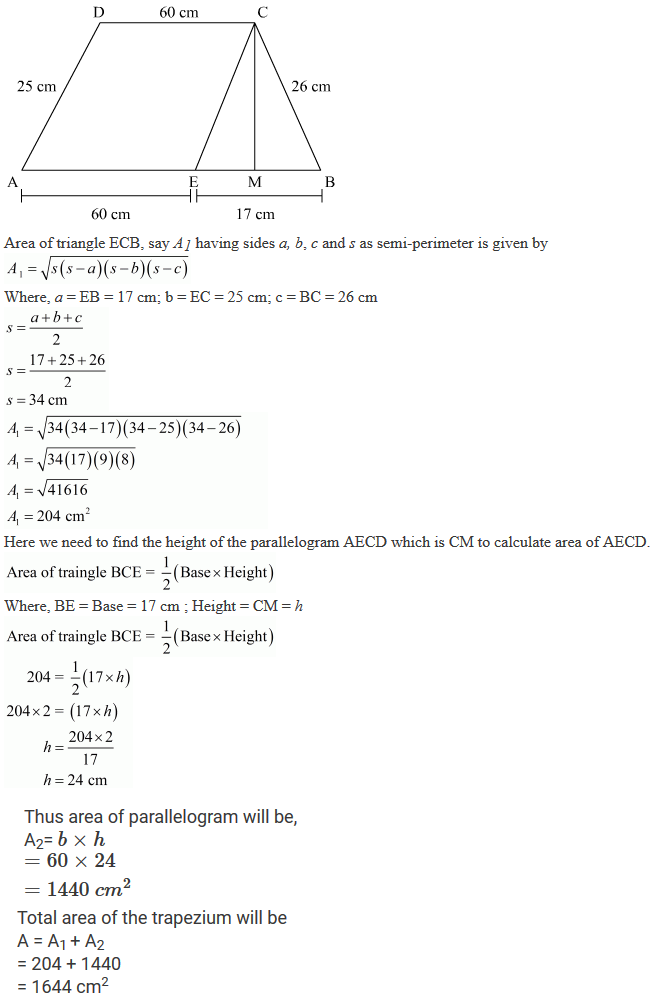6. Find the area of a rhombus whose perimeter is 80 m and one of whose diagonal is 24 m.

Solution

We assume ABCD be the given rhombus having
AB = BC = CD = DA
Diagonal DB divides rhombus into two equal triangles BDC and ADB7. A rhombus, sheet, whose perimeter is 32 m and whose one diagonal is 10 m long, is painted on both sides at the rate of Rs 5 per m2. Find the cost of painting.

Solution

We assume ABCD be the given rhombus having
AB = BC = CD = DA
BD and AC be the diagonals of rhombus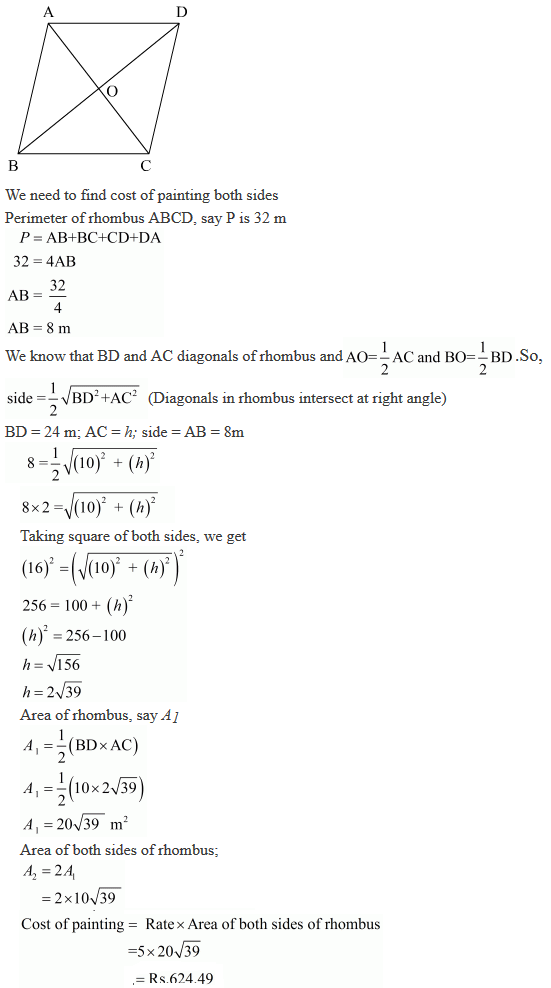8. Find the area of a quadrilateral ABCD in which AD = 24 cm, ∠BAD = 90° and BCD forms an equilateral triangle whose each side is equal to 26 cm.

Solution

We assume ABCD be the quadrilateral having sides AB, BC, CD, DA, diagonal BD and ∠BAD = 90° where BCD forms an equilateral triangle having equal sides.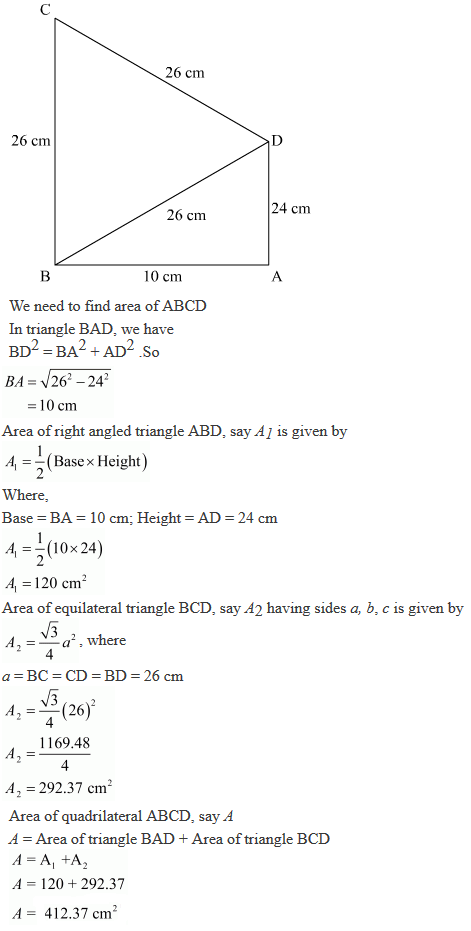9. Find the area of a quadrilateral ABCD in which AB = 42 cm, BC = 21 cm, CD = 29 cm, DA = 34 cm and diagonal BD = 20 cm.

Solution

The quadrilateral ABCD having sides AB,BC,CD,DA and diagonal BD is given, where BD divides ABCD into two triangles
ΔDBC and ΔDAB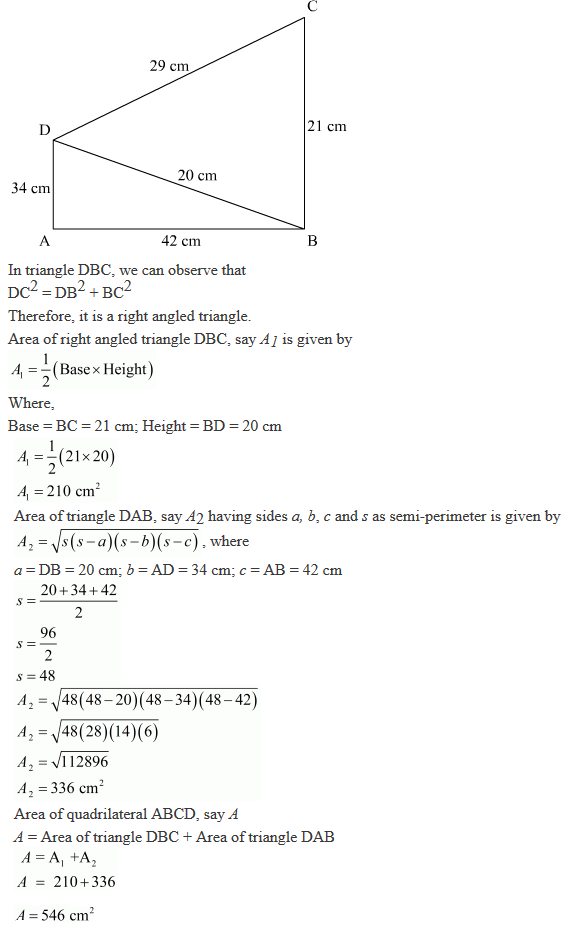10. Find the perimeter and area of the quadrilateral ABCD in which AB = 17 cm, AD = 9 cm, CD = 12 cm, ∠ACB = 90° and AC = 15 cm.

Solution

We assume ABCD be the quadrilateral having sides AB, BC, CD, DA and ∠ACB = 90.
We take a diagonal AC, where AC divides ABCD into two triangles ΔACB and ΔADC11. The adjacent sides of a parallelogram ABCD measure 34 cm and 20 cm, and the diagonal AC measures 42 cm. Find the area of the parallelogram.

Solution

We are given the measure of adjacent sides of a parallelogram AB and BC that is the sides having same point of origin and the diagonal AC which divides parallelogram ABCD into two congruent triangles ABC and ADC.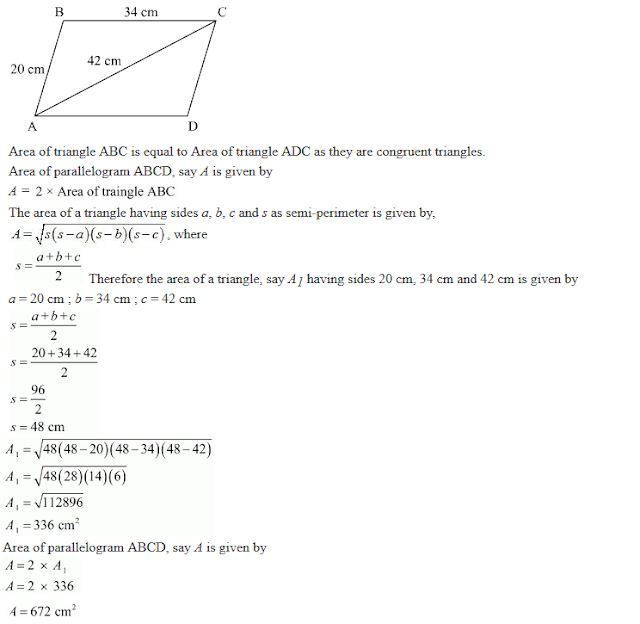12. Find the area of the blades of the magnetic compass shown in Fig. 12.27 (Take √11 = 3.32).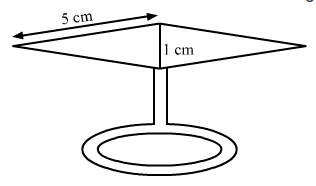Solution